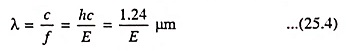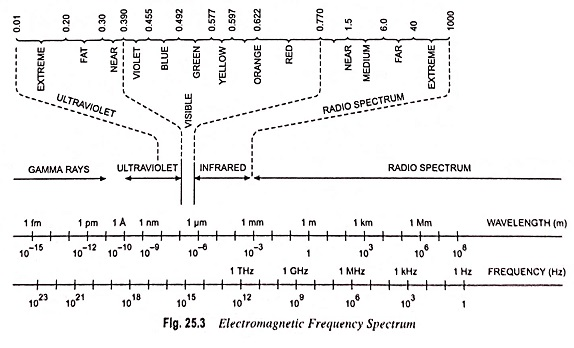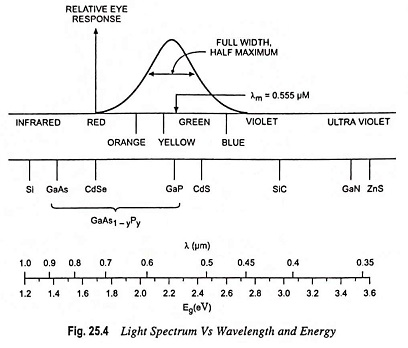## Optical Absorption in Electronics:

As discussed in Art. 25.3, light waves can be treated as particles, which are referred to as photons. The energy of a photon is E = hf where h is Plank’s constant and f is the frequency. Wavelength and energy can be related bywhere E is the photon energy in eV and c is the velocity of light.

Photons can interact with

• impurity atoms, donors or acceptors,
• defects within the semiconductors.

Interaction with valence electrons is of more interest as these collisions generate sufficient energy to elevate electrons into the conduction band. This further generates electron-hole pairs (EHP) and produces excess carrier concentrations.

Photons with an energy larger than the band gap energy are absorbed by materials. Where the valence band contains many electrons and the conduction band has many empty states the photon absorption is higher when the electrons may be excited.

Three situations normally arise with photons energy conditions viz.

• an electron excited to the conduction band by optical absorption initially has more energy than the conduction band electrons (unless heavily doped) which enables scattering of energy to the lattice.
• A photon with energy less than the conduction band gap energy EG cannot excite the electron from the valence band to the conduction band. Thus in a pure semiconductor there is negligible optical absorption of photons with hf < EG. This explains why some materials are transparent in certain wavelength ranges.
• When a beam of photons with energy greater than the conduction band gap energy (hf > EG) falls on a semiconductor, a predictable amount of optical absorption will occur depending on the properties of the material.Figures 25.3 and 25.4 give the band gap energy of some semiconductors and details the visible, infrared and ultraviolet part of the spectrum

• Infrared ranges—GaAs, Si, Ge, InSb
• Visible range—GaP, CdS
• Ulteraviolet range—ZnS, SiC

GaP and CdS have band gaps wide enough to pass photons in the visible range. A semiconductor absorbs photons with energies equal to the band gap or larger. Silicon (Si) absorbs not only band gap light (~ 1 μm) but also shorter wavelengths, including those in the visible part of the spectrum.

Scroll to Top